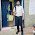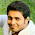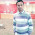Dear students,

Numerical Ability for SBI Clerk Prelims 2018

Today is the Day 44 of the SBI Clerk 60 Days Study PlanNumerical Ability Section has given heebie-jeebies to the aspirants when they appear for a banking examination. As the level of every other section is only getting complex and convoluted, there is no doubt that this section, too, makes your blood run cold. The questions asked in this section are calculative and very time-consuming. But once dealt with proper strategy, speed, and accuracy, this section can get you the maximum marks in the examination. Following is the Numerical Ability quiz to help you practice with the best of latest pattern questions.

Q1.  When an article is sold at 4/5th of its selling price, there is a loss of 28%. What will be profit percentage, when the same article is sold at 6/5th of the its selling price?
(a) 100/9%
(b) 8%
(c) 10%
(d) 25/2%
(e) 15%

Q2.  If a discount of 30% given on the marked price of an article, the shopkeeper gets a profit of 5%. Find his percent loss, when he allows two successive discounts of 36% and 25% on the marked price?
(a) 20%
(b) 25%
(c) 30%
(d) 15%
(e) 28%

Q3.  A trader bought two horses for Rs. 8,400. He sold first horse at 4% loss while second horse at 17% profit, if his overall gains was Rs. 420. Find the cost price of the horse, which sold at 17% profit.
(a) Rs. 3,600
(b) Rs. 4,000
(c) Rs. 3,250
(d) Rs. 2,700
(e) Rs. 4500

Q4.  Sumit purchased two watches for Rs. 17334. He sold first watch at 6% loss and second at 8% profit and found that the loss amount is equal to the profit amount. Then, find the difference between cost price of both watches.
(a) Rs. 142
(b) Rs. 162
(c) Rs. 212
(d) Rs. 192
(e) Rs. 172

Solutions (1-5):

Q6. A shokeeper bought 150 calculators at the rate of Rs. 250 per calculator. He spent Rs. 2500 on transportation and packing. If the marked price of calculator is Rs. 320 per calculator and the shopkeeper gives a discount of 5% on the marked price, then what will be the profit percentage of the shopkeeper?
(a) 20%
(b) 14%
(c) 15%
(d) 16%
(e) 18%

Q7.  Mr. Usman bought two goat each for Rs. 6,600. He sold both goats to Mr. Razak, one at a loss of 16 ⅔% and other at a profit of 33⅓%. Find his overall profit/loss percentage in this transaction.
(a) 6%
(b) 25/3%
(c) 16/3%
(d) 20/3%
(e) 12%

Q8.  The marked price of an LED TV is 66⅔% more than its cost price. This LED TV is sold to a person at a discount of 16 ⅔% on marked price. If selling price of LED TV is Rs. 4250 then find its cost price.
(a) Rs. 3060
(b) Rs. 3600
(c) Rs. 3006
(d) Rs. 2860
(e) Rs. 3040

Q9.  A shopkeeper has 80 kg pure sugar. He mixes a certain quantity of salt (in kg) in it and made profit of 25% by selling the mixture at its cost price. If cost price of pure sugar was Rs. 24 per kg then how much amount of salt the shopkeeper mixed to the pure sugar (Assume cost of salt to be zero)?
(a) 10 kg
(b) 25 kg
(c) 20 kg
(d) 24 kg
(e) 40 kg

Q10.  A person bought 9 oranges for Rs. 24 and sells these oranges at a rate of Rs. 54 per dozen. Find his overall profit percentage.
(a) 65.78%
(b) 67.85%
(c) 62.75%
(d) 68.75%
(e) 64.75%

Solutions (6-10):

Q11. Ratio between marked price and cost price of an article is 5 : 3. If this article is sold at Rs. 60 a profit of 25% is obtained. If the same article is sold at its marked price then the profit percentage will be:
(a) 100/3%
(b) 200/3%
(c) 50/3%
(d) 48%
(e) 54%

Q12. Two friends bought two different lands at a price of Rs. 4000 and Rs. 6000 respectively. The value of first land increases 25% per year and second land increases at 5% per year. At the end of two year they exchange the land. Find profit % of gainer.
(a) 6.12%
(b) 5.84%
(c) 5.46%
(d) 6.24%
(e) 5.64%

Q13. Veer bought some chairs and tables from a shopkeeper which are in the ratio of 9 : 8. Marked price of a chair and a table is in the ratio of 5 : 7. Shopkeeper gives discount of 20% and 25% on chairs and tables respectively. If total discount offered is 4600 Rs. Find the total selling price of chairs
(a) 6000 Rs.
(b) 4800 Rs.
(c) 3600 Rs.
(d) 7200 Rs.
(e) 5000 Rs.

Q14. A shopkeeper allows 4% discount and allows two articles free on purchase of fourteen articles. He earns 40% profit during the transaction. By what above the cost price he marked goods?
(a) 35%
(b) 100/3%
(c) 25%
(d) 20%
(e) 200/3%

Q15. If a shopkeeper offers a single discount of 25% instead of two successive discounts of 10% and 15%, he loses Rs.30 on selling an article. If the price of the article is marked up by 60%, then what is the cost price of the article?
(a) Rs.2000
(b) Rs.1250
(c) Rs.1500
(d) Rs.1000
(e) Rs. 1225

Solutions (11-15):1.4th is wrong

2.7 question solve in ratio method??

3.Plz explain question 1... How 72 came in denominator while finding cp

4.Plz explain question 1... How 72 came in denominator while finding cp

5.28 percent loss hai islie 72 in 100

6.Thank you... Also I think question 4 is wrong

7.Plz explain qn no 13

8.9.ya que 4 ka sol v wrong diya h becoz 2nd sp me 1st sp ko minus kar raha h while 2nd part me (17334-x) minus hona chahiye tha